### Home > CALC > Chapter 7 > Lesson 7.3.2 > Problem7-124

7-124.
1. Differentiate the following equations. Homework Help ✎

1.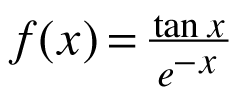2. y3 − 3xy = 3y

3. g(t) = ln(t)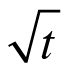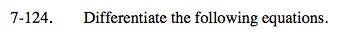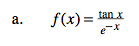You could use the Quotient Rule, or rewrite this fraction without the negative exponent in the denominator.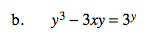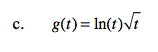Before differentiating, rewrite g(t) with a fractional exponent.